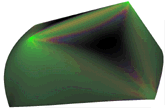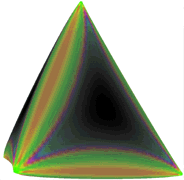# Scaling properties of stretching ridges in a crumpled elastic sheet

Alex Lobkovsky, James Franck Institute, University of Chicago, Chicago IL 60637.

Sharon Gentges

Hao Li, Present address: NEC Research Institute, 4 Independence Way, Princeton, NJ 08540

David Morse, Institute for Theoretical Physics, University of California, Santa Barbara CA 93106.

T.A. Witten

### ABSTRACT of paper accepted in Science 9/95

Strong deformation of a sheet of solid material often leads to a crumpled state having sharp points of high curvature. A scaling property governing the crumpled state has been numerically demonstrated by examining the {\it ridges} joining pairs of sharp points in a range of simple geometries of variable size. As the linear size $X$ increases sufficiently, the deformation energy grows as $X^{1/3}$. Remarkably, it consists of similar amounts of bending and stretching energy. This energy becomes concentrated in a fraction of the sheet that decreases as $X^{-1/3}$. Despite this concentration, the local strain in the ridge decreases, as $X^{-2/3}$. Nearly all the deformation energy in thin, crumpled elastic sheets should be concentrated in ridges that obey these scaling laws.

•Energy distribution in a numerically-modeled, flat sheet of elastic material from which two 60-degree wedges were cut. The cut edges were then joined to form a boat shape showing a ridge singularity. Distance between vertices is 1377 times the thickness. Color indicates ratio of stretching to bending energy. Near the vertices bending energy is more than ten times stretching energy. In the middle of the ridge bending energy is 4.9 times stretching energy. Alternating color stripes flanking the ridge are caused by the vanishing of first stretching energy, then bending energy as one moves away from the ridge. Faint induced ridges extend from each vertex and meet at the edges at front and back. Similar ridge structures occur when a sheet or membrane is crumpled.
•Energy distribution in a thin, flat, sheet of elastic material, cut and joined to form a tetrahedron, showing ridge singularities. Distance between vertices is 1581 times the thickness. Color indicates ratio of stretching to bending energy. Near the vertices, bending energy is more than ten times stretching energy. In the middle of the ridge bending energy is 2.95 times stretching energy. Brightness indicates total energy density. Alternating color stripes flanking the ridges are caused by the vanishing of first stretching, then bending energy as one moves away from the ridge. Similar ridge structures occur when a sheet or membrane is crumpled. Colored patches in the faces near each vertex are artifacts from the lattice used to simulate the sheet.
• Stretching-energy profile of tetrahedron. Darkness is stretching energy density
University of Chicago Materials Center